Oscillations• Simple harmonic motion
• Oscillator vs. wave
• Simple harmonic oscillators
• Mass on a spring
• Pendulum
• Physical pendulum
• Energy
• Damping
• Driven oscillations
• Resonance

Simple harmonic motion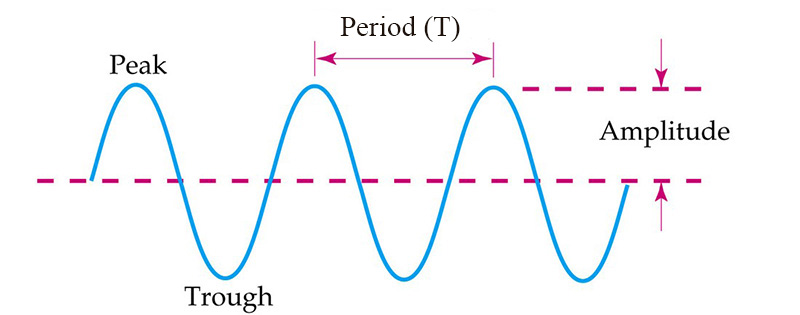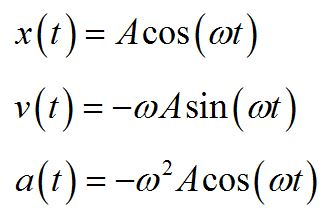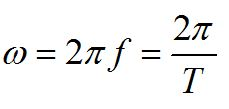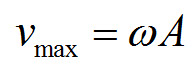An undamped mass oscillating on a spring produces a sine wave. Note that a maximum or minimum value of position corresponds with a zero in velocity. Acceleration is not constant, but varies out of phase with position vs. time.

Practice problems

A block of mass m is on a frictionless surface as shown, attached to an ideal spring. The spring is initially unstretched at length x0. The block is pulled to the right to the position xf as shown and released. The block then makes 12 oscillations in 7.0 seconds.

Consider the case where m = 0.18 kg, x0 = 0.35 m and xf = 0.48 m.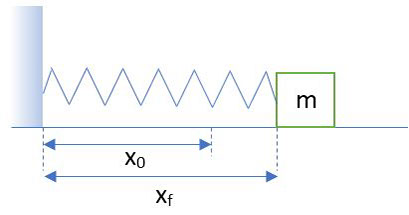1. What is the position of the block at 3.5 s?

2. What is the velocity of the block at 3.5 s?

Rotation and simple harmonic oscillation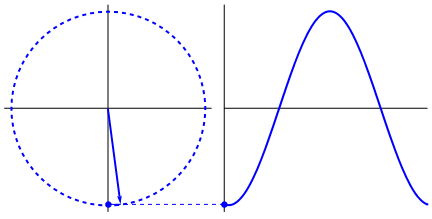A rotating object can also be considered a simple harmonic oscillator. Its projection produces a sine wave.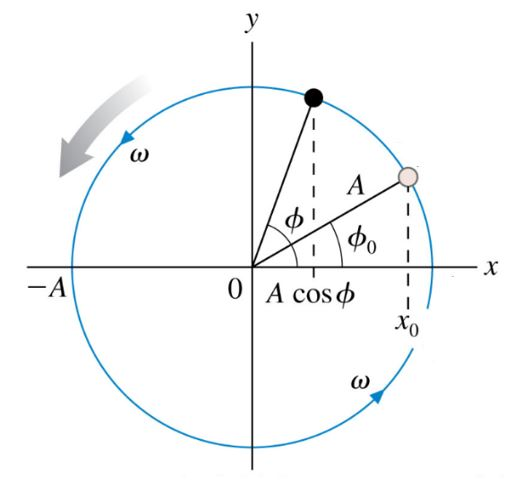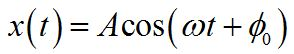The phase is the argument of the sine function. The phase constant provides initial conditions for the oscillation, when t = 0.

Simple harmonic oscillator: mass on a spring

Practice questions

1. Consider a simple harmonic oscillator: mass on a spring. If you increase the mass, what happens to the frequency of oscillation?

2. Consider a simple harmonic oscillator: mass on a spring. If you increase the stiffness of the spring, what happens to the frequency of oscillation?

3. Consider a simple harmonic oscillator: mass on a spring. If you increase gravity, what happens to the frequency of oscillation?